# Constructive Solid Geometry and Function Representation in R

Version 1: Monday 19 December 2022The HyperFun Project provides a language and interpreter for describing 3D scenes using Function Representation and Constructive Solid Geometry. This document describes the R package 'hyperfun', which provides an interface to the HyperFun language and interpreter.

## 1. Introduction

Constructive solid geometry (CSG) is a technique for creating complex three-dimensional shapes by combining simple three-dimensional shapes. For example, the shape shown on the right (below) is a complex shape that would be difficult to describe directly, but is easily produced by "subtracting" the simple sphere shown in the middle from the simple cube shown on the left.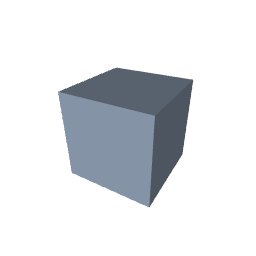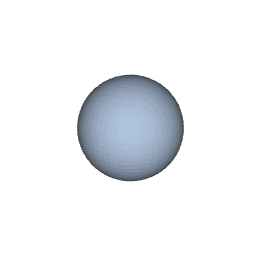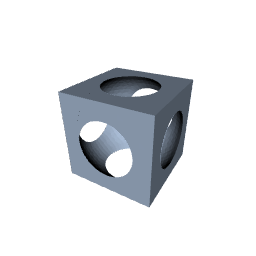The HyperFun project (Adzhiev et al., 2002) defines a language for describing and combining simple shapes and provides a program, hfp, which is an interpreter for the HyperFun language and a viewer for the resulting three-dimensional image. For example, the following HyperFun code describes a sphere (centred at the origin, with a radius of 7), a cube (centred at the origin, with a width, height, and depth of 12), and an overall model that consists of the sphere subtracted from the cube.

  my_model(x, a)
{
array center;
array vertex;
center = [0, 0, 0];
sphere = hfSphere(x, center, 7);
vertex = [-6, -6, -6];
cube = hfBlock(x, vertex, 12, 12, 12);
my_model = cube \ sphere;
}


If the code above is saved in a file called csg.hf, the following command runs the code and opens a window to view the resulting image.

hfp csg.hf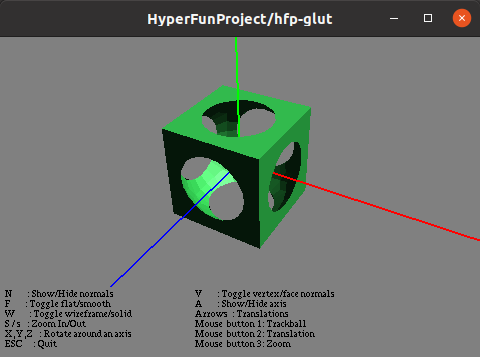The 'hyperfun' package provides an R interface to HyperFun so that R users can write idiomatic R code to generate, and view, complex three-dimensional shapes using the HyperFun system. For example, the following R code describes a sphere (with a radius of 7), a cube (with width, height, and depth of 12), and creates a model consisting of the sphere subtracted from the cube.

library(hyperfun)

sphere <- hfSphere(r = 7)
cube <- hfBlock(dx = 12, dy = 12, dz = 12)
model <- cube - sphere


The hfp() function calls the HyperFun program hfp to calculate the resulting image and then draws the image using the 'rgl' package (Murdoch and Adler, 2022).

hfp(model)Clearly, the headline act in this drama is the HyperFun system. However, the 'hyperfun' package makes three useful contributions:

The convenience of the R packaging system means that the 'hyperfun' package is an easy way for R users to install and run HyperFun across multiple platforms.

2. A convenient and familiar interface to HyperFun for R users.

The 'hyperfun' package allows R users to write R code to create HyperFun models; they do not have to learn the HyperFun language.

The Section on The 'hyperfun' package gives an overview of the R functions provided by the 'hyperfun' package for creating HyperFun models.

3. An extension of HyperFun to include R functions.

The 'hyperfun' package provides an hfR() function so that HyperFun objects can be defined using R functions. This access to R makes it possible to express a much wider range of HyperFun models than is possible with the original HyperFun language.

The Section on Function representation describes this extension of HyperFun in more detail.

## 2. The 'hyperfun' package

In basic usage of the 'hyperfun' package, we only need to make use of functions that define objects (three-dimensional shapes) and operators that can be used to combine objects.

## 3D objects

We have already seen two functions that define objects, hfSphere() for spheres and hfBlock() for rectanguloid objects. In both cases, there are arguments to control the placement and size of the objects. For example, the following code defines a sphere centred at (0, 0, 0), with radius 7.

sphere <- hfSphere(cx = 0, cy = 0, cz = 0, r = 7)


The print() method for objects shows the HyperFun code that the object generates and the hfp() function draws the object in an 'rgl' window.

sphere

  HF_9_1(x, a)
{
array center;
center = [0, 0, 0];
sphere = hfSphere(x, center, 7);
HF_9_1 = sphere;
}

hfp(sphere)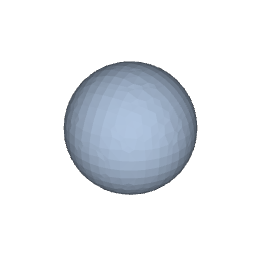The following table lists the shapes that are currently available in 'hyperfun', along with the relevant function and sample output.

 Rectanguloid hfBlock(x, y, z, dx, dy, dz, jx, jy, jz)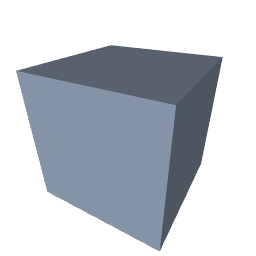jx is justification (0 to 1). Sphere hfSphere(cx, cy, cz, r)Ellipsoid hfEllipsoid(cx, cy, cz, a, b, c)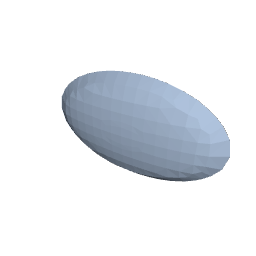a is length of semi-axis in X dimension. Cylinder hfCylinder(cx, cy, cz, r, axis)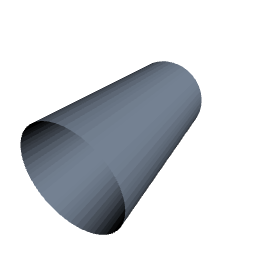axis specifies the cone axis, e.g., "X". Elliptic cylinder hfEllCylinder(cx, cy, cz, a, b, axis)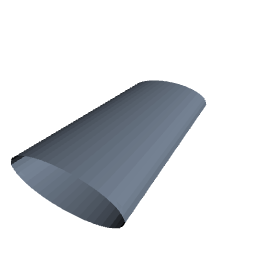See meaning of parameters for cones and ellipsoids. Cone hfCone(cx, cy, cz, r, axis)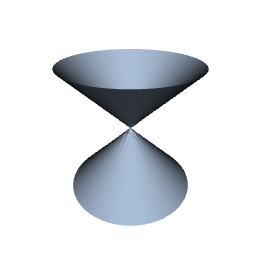r is cone radius at height 1. Elliptic cone hfEllCone(cx, cy, cz, a, b, axis)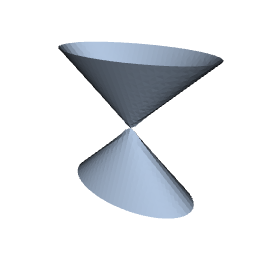See meaning of parameters for cones and ellipsoids. Torus hfTorus(cx, cy, cz, R, r0, axis)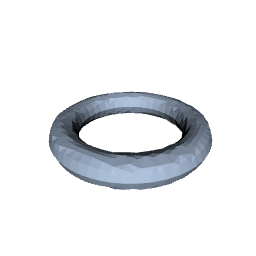R is radius of revolution; r0 is radius of disk. Super ellipsoid hfSuperEll(cx, cy, cz, a, b, c, s1, s2)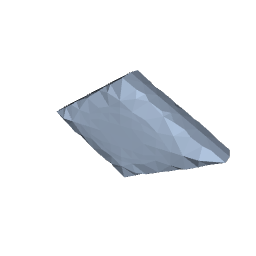s1 controls "sharpness" in z-direction; s2 controls "sharpness" in xy-direction. Blobby object hfBlobby(x0, y0, z0, a0, b0, T)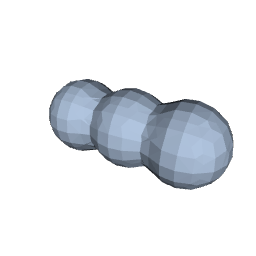x0 specifies X centres of blobs; a0 and b0 and T control how connected the blobs are.

## 3D operators

Objects can be combined using arithmetic and logical operators. For example, the following code subtracts an inverted sphere from a cube; rather than carving a hole out of the centre of the cube, this chops the corners off the cube. Again, the print() method shows the corresponding HyperFun code and hfp() draws the resulting image.

model <- cube - !sphere
model

  HF_7_1(x, a)
{
array vertex;
vertex = [-6, -6, -6];
block = hfBlock(x, vertex, 12, 12, 12);
HF_7_1 = block;
}
HF_9_1(x, a)
{
array center;
center = [0, 0, 0];
sphere = hfSphere(x, center, 7);
HF_9_1 = sphere;
}
HF_31_1(x, a)
{
HF_31_1 = ~HF_9_1(x, a);
}
HF_32_1(x, a)
{
HF_32_1 = HF_7_1(x, a) \ HF_31_1(x, a);
}
my_model(x, a)
{
my_model = HF_32_1(x, a);
}

hfp(model)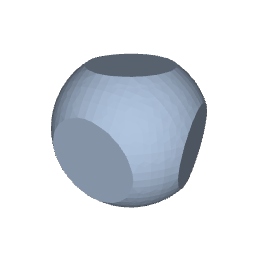The list below shows the set of available operators:

• The | operator (vertical bar; logical or) produces the union of two objects.
• The & operator (ampersand; logical and) produces the intersection of two objects.
• The - operator (minus) subtracts one object from another.
• The ! operator (exclamation; logical not) inverts an object so that the interior of the object becomes its exterior.

### Vectorised 3D objects

The arguments to functions that define objects, like hfSphere() and hfBlock(), can be vectors, so it is easy to define several objects at once. For example, the following code defines eight spheres arranged in a circle.

t <- seq(0, 2*pi, length.out=9)[-1]
x <- 7*cos(t)
z <- 7*sin(t)
spheres <- hfSphere(cx = x, cz = z, r = 2)

hfp(spheres)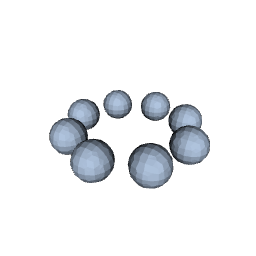### The hfp() function

The spheres in the image above are quite coarse; there are fewer facets on each of the spheres. We can provide arguments to the hfp() function to increase the resolution of the final image. For example, the following code draws the multiple spheres using a higher density value (the default is 30), which produces much smoother spheres.

hfp(spheres, density = 100)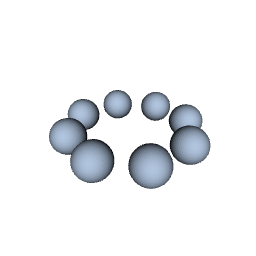Another parameter that affects the final image is the bounding box used by the HyperFun system. There is a bbox argument to hfp() to control this bounding box. For example, the following code draws the multiple spheres again, but with a bounding box that cuts the spheres in half. The default bounding box has limits from -10 to 10 in each dimension, but this code sets the limits in the y-dimension from -5 to 0.

hfp(spheres, density = 100, bbox = c(-10, -5, -10, 10, 0, 10))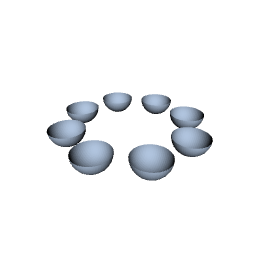By default, hfp() function draws the final image in an 'rgl' window, but we can instead produce an output file if we specify a format value. For example, the following code generates an STL file; possible formats are "stl" for STL, "stlb" (STLB; binary STL), "pov" (POV-Ray), "cli" (CLI), and "wrl" (VRML). The default format that draws on screen is "rgl".

stlFile <- hfp(hfSphere(), format="stl")


The name of the STL file that is generated is returned by hfp().

stlFile

   "/tmp/RtmpWYs49y/file47115b9127.stl"


Any further arguments are passed to rgl::readSTL() (when format="rgl"). For example, the following code specifies a surface colour and a specular colour for rendering.

hfp(hfSphere(), color="blue", specular="black")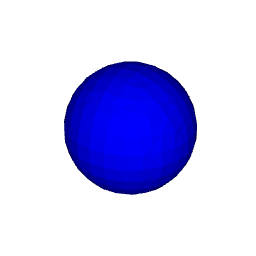### The hfModel() function

When we combine objects using one of the arithmetic or logical operators, the result is a model. We can also explicitly create a model using the hfModel() function, which takes one or more objects and an operator to combine them. For example, the following two expressions produce exactly the same result.

cube - sphere
hfModel(cube, sphere, op="-")


A model can also consist just of a single object, as shown below.

hfModel(cube)

  HF_7_1(x, a)
{
array vertex;
vertex = [-6, -6, -6];
block = hfBlock(x, vertex, 12, 12, 12);
HF_7_1 = block;
}
HF_43_1(x, a)
{
HF_43_1 = HF_7_1(x, a);
}
my_model(x, a)
{
my_model = HF_43_1(x, a);
}


When we define multiple objects at once and create a model that combines those multiple objects with another object, the multiple objects are first "reduced" to a single model that consists of the union of the multiple objects. For example, the following code subtracts the cube from earlier examples from the eight spheres. The eight spheres are first "reduced" to the union of the eight spheres and then the cube is substracted.

hfp(spheres - cube, density = 100)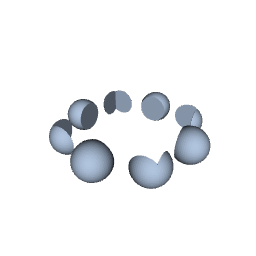If we want to reduce multiple objects to a single model using a different operator, we can use the hfModel() function. For example, the following code draws two spheres that overlap with each other.

twoSpheres <- hfSphere(c(-2, 2), c(0, 1), c(0, 1), r=2.5)

hfp(twoSpheres)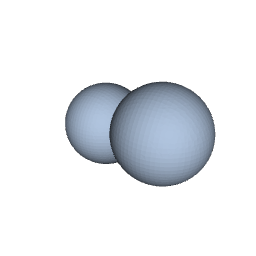The following code uses the - (minus) operator to reduce the multiple spheres, which leaves one sphere with a bite taken out of it (or a "death star" if you are that way inclined).

spheresMinus <- hfModel(twoSpheres, op="-")

hfp(spheresMinus)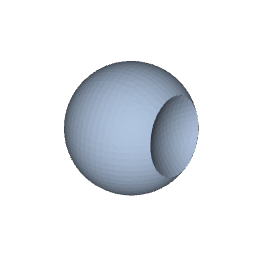The hfModel() function can also be used to combine more than two objects or models at once. For example, the following code subtracts our original sphere from our original cube and then also subtracts the circle of smaller spheres from the result.

threesome <- hfModel(cube, sphere, spheres, op="-")

hfp(threesome)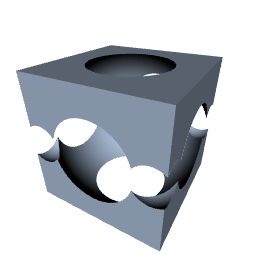### Evaluating raw HyperFun code

We can give the hfp() function a character vector as the first argument, in which case the character values are assumed to be raw HyperFun code. The character values are evaluated using hfp and the result is drawn with 'rgl'. For example, suppose we have a character vector called hfCode that contains the raw HyperFun code from the very first example in the introduction.

hfCode

    "my_model(x, a)"
 "{"
 "  array center;"
 "  array vertex;"
 "  center = [0, 0, 0];"
 "  sphere = hfSphere(x, center, 7);"
 "  vertex = [-6, -6, -6];"
 "  cube = hfBlock(x, vertex, 12, 12, 12);"
 "  my_model = cube \\ sphere;"
 "}"

hfp(hfCode)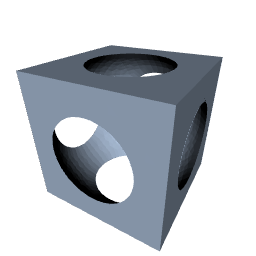We can also provide just a single character value as the first argument to hfp(), in which case the value is interpreted as the name of a file that contains HyperFun code. For example, the following code produces the same sphere result.

writeLines(hfCode, "csg.hf")

hfp("csg.hf")## 3. Function representation

All of the HyperFun examples so far have made use of predefined HyperFun functions such as hfSphere() and hfBlock(). These predefined functions are known as the FRep library. However, this FRep library is actually just a convenience layer on top of a much more general and powerful idea: Function Representation (FRep).

In the HyperFun system, a three-dimensional solid is defined by a function of three variables (x, y, z); conceptually, the function is evaluated at all values of (x, y, z) and regions that produce a negative value are inside the solid, regions that produce a positive values are outside the solid, and the boundary, where the function returns zero, defines the surface of the solid. For example, the following HyperFun code defines a paraboloid. For any (x, y, z) coordinate, the model returns y minus the sum of half-x squared and half-z squared. This creates a surface wherever that expression is zero, which describes the paraboloid $y = (x/2)^2 + (z/2)^2$.

  my_model(x, a)
{
my_model = x - (x/2)^2 - (x/2)^2;
}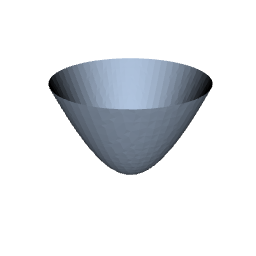### The hfR() function

The 'hyperfun' package provides functions such as hfSphere() and hfBlock() that correspond to functions in the HyperFun FRep library. In addition, the 'hyperfun' package provides an hfR() function that allows the user to define a HyperFun object in terms of an R function. The argument to hfR() is a function that should accept a single argument, which is a numeric vector of three values, and it should return a single numeric value.

This means that with 'hyperfun' we can express three-dimensional objects using FRep, by writing an R function. For example, the following code defines a paraboloid.

f <- function(xyz) xyz - (xyz/2)^2 - (xyz/2)^2
paraboloid <- hfR(f)

hfp(paraboloid)The downside to using hfR() is that the R function gets evaluated many times for many different xyz values, so this is slower than coding directly in HyperFun. On the other hand, being able to write an R function means that we have access to the full generality of the R language and all of its extension packages. This greatly expands the sort of surface that we can easily express in FRep. For example, the following code draws a multivariate normal surface using the dmvnorm() function from the 'mvtnorm' package (Genz and Bretz, 2009).

library(mvtnorm)
mvnorm <- hfR(function(xyz) xyz - 20*dmvnorm(sqrt(sum((xyz[c(1, 3)]/2)^2))))

hfp(mvnorm)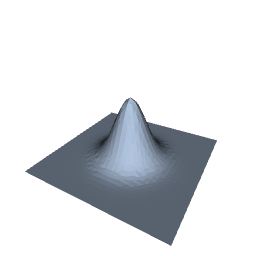The hfR() function also provides an easier way for users to extend the FRep library. Adding a new shape to the FRep library itself requires writing C code and compiling a new version of hfp. With hfR(), and the general extensible nature of R, users are able to write their own hf*() function and share it with others easily. For example, the following code defines a new hfParaboloid() function with parameters to control the curvature in both x and z dimensions.

hfParaboloid <- function(a = 1, b = 1) {
f <- function(xyz) {
xyz - sign(a)*(xyz/a)^2 - sign(b)*(xyz/b)^2
}
hfR(f)
}


With that function defined, anyone can easily create a variety of paraboloids. For example, the following code draws a saddle-shaped paraboloid.

saddle <- hfParaboloid(2, -2)

hfp(saddle)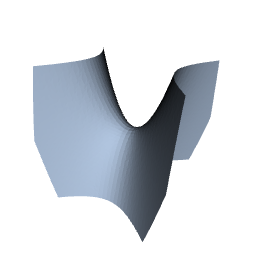## 4. Applications

The 'hyperfun' package is useful for describing 3D objects in R. The ability to describe an object using FRep and the ability to describe an object in terms of intersections and unions of other objects makes it easier to describe more complex 3D objects. But what use are 3D objects in R? The established guidelines for data visualisations (Cleveland, 1985; Cleveland, 1993; Ware, 2012) advise against the use of 3D objects to convey information from data. Are there any valid (statistical [graphics]) applications for 3D objects in R?

One possible application is statistical diagrams to help explain mathematical algorithms. For example, the diagram below might be used to help visualise the solution space based on a set of three linear constraints. The planes representing the linear constraints can be expressed mathematically using FRep and the solution space is naturally expressed by intersecting the three planes using CSG.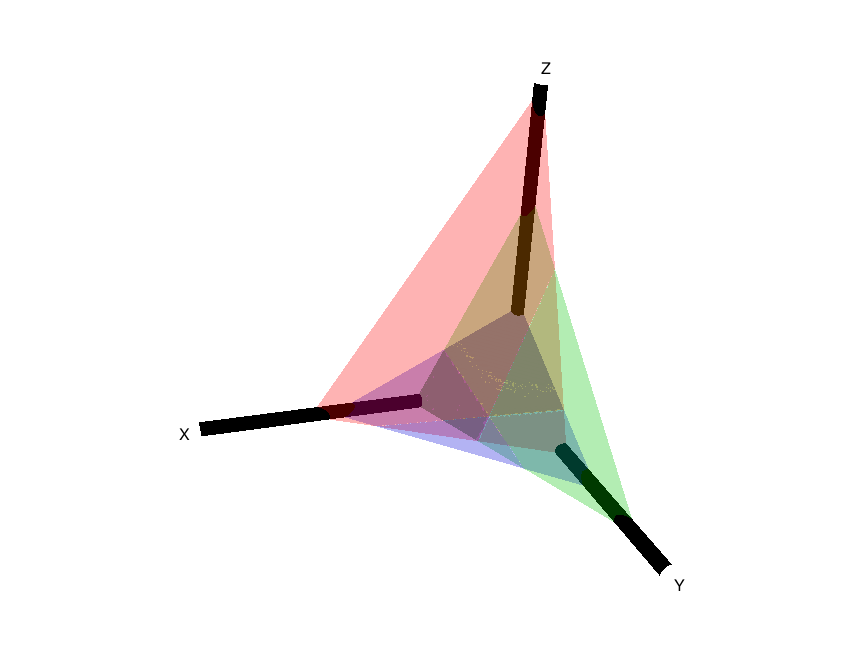Of course, 'hyperfun' does not have to be used for statistical applications. Given a 3D printer, we can even use it to produce real world objects, such as the dice below.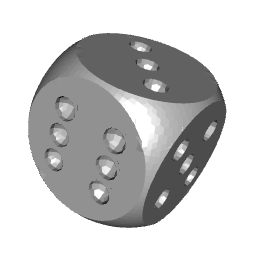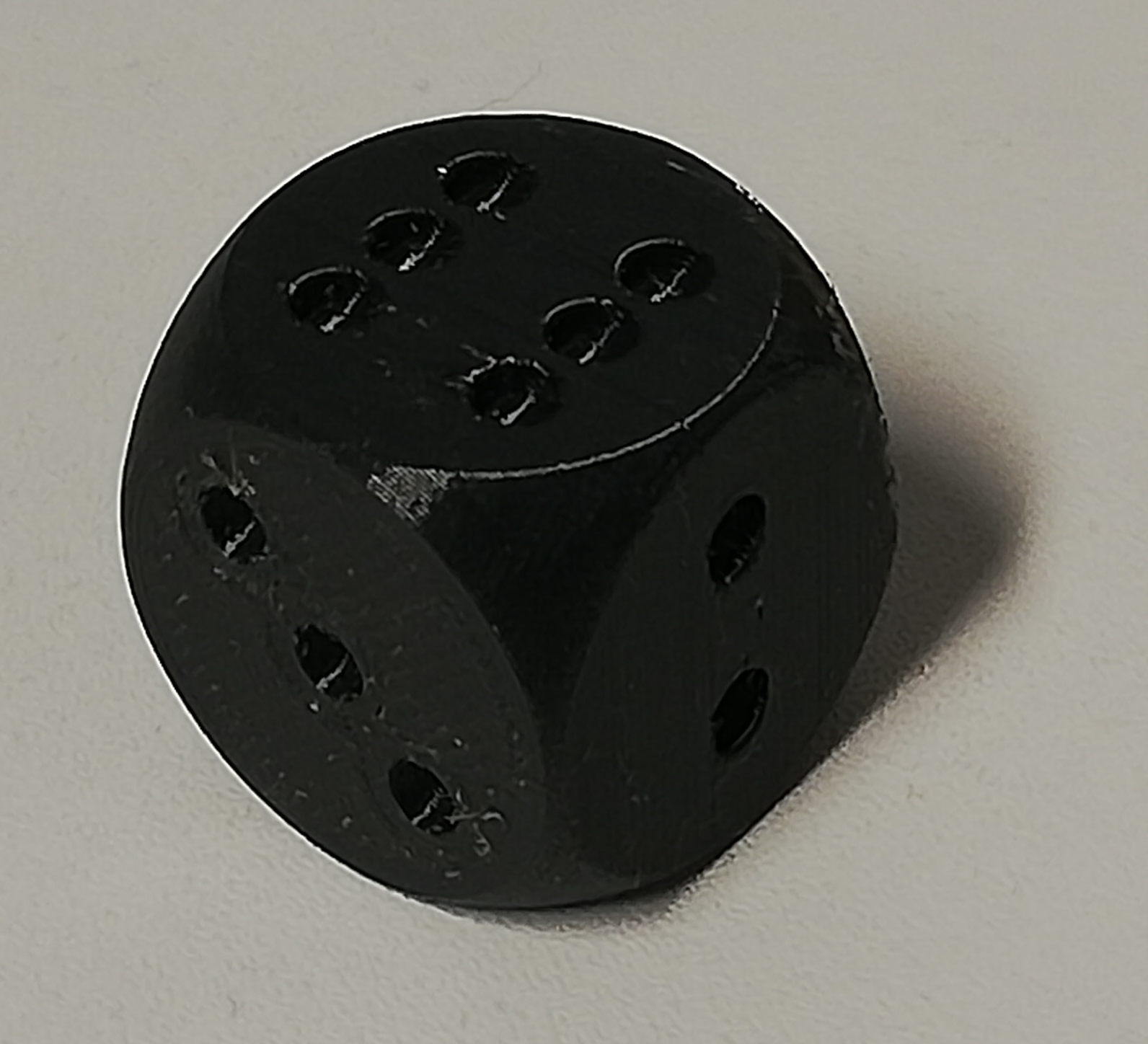## 5. Discussion

The HyperFun Project provides a language and interpreter for describing 3D scenes that is very powerful and convenient because it harnesses both FRep and CSG. However, there are several limitations:

• The HyperFun language has limitations. For example, it supports mathematical functions like sqrt, log, and sin, but only a small set of such functions.

• The FRep library is difficult to extend. Adding new FRep functions requires writing C code and recompiling the HyperFun interpreter.

• The official HyperFun web site, hyperfun.org, has been abandoned. A copy of the web site is still available at http://paulbourke.net/dataformats/hyperfun/, but that is also a little out of date and does not contain as many examples of HyperFun programs as the official web site had. On the other hand, the HyperFun source code is still available from SourceForge.

The 'hyperfun' package for R provides an easy way (for R users) to access, install, and run the HyperFun interpreter. It also provides a convenient interface (for R users) to generate 3D models in the HyperFun language. Furthermore, the 'hyperfun' package, with its hfR() function, provides a generalised extension mechanism for the HyperFun language. We can describe 3D shapes using FRep simply by writing R functions. This combines the full power, flexibility, and extensibility of R with the HyperFun Project.

### Resolution and speed

The description of a 3D model in HyperFun code is a pure, typically continuous, mathematical description. However, the generation of 3D output from a HyperFun model, whether on screen or by writing to an external file, involves the construction of a finite polygon mesh from the HyperFun model. The HyperFun interpreter constructs a polygon mesh by evaluating the HyperFun model at locations on a regular grid in 3D space. The bounds of this grid are controlled by the bbox argument to the hfp() function and the resolution of the grid is controlled by the density argument.

Some care is required to make sure that the resolution of the regular grid is sufficiently high to capture all details of 3D objects. Even something as simple as a plane can be represented poorly or even disappear in the final rendering if the plane is aligned with one of the main axes, but is not aligned with the regular grid.

Generating 3D output requires at least one evaluation for each model at each location on the regular grid, so more complex models, involving more 3D objects, and higher resolution scenes require a larger number of evaluations. More evaluations means a longer run time and the increase is roughly linear in terms of number of 3D objects and cubic in terms of grid density, as shown in the plots below.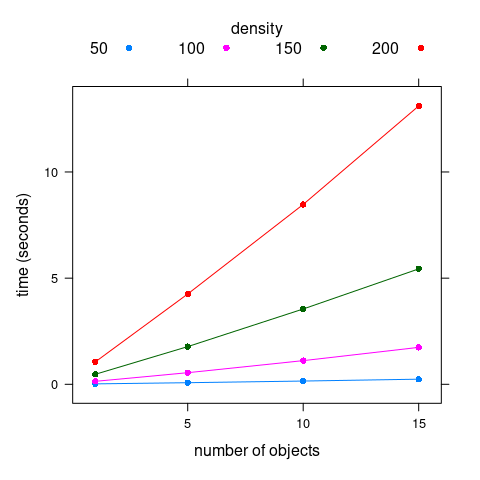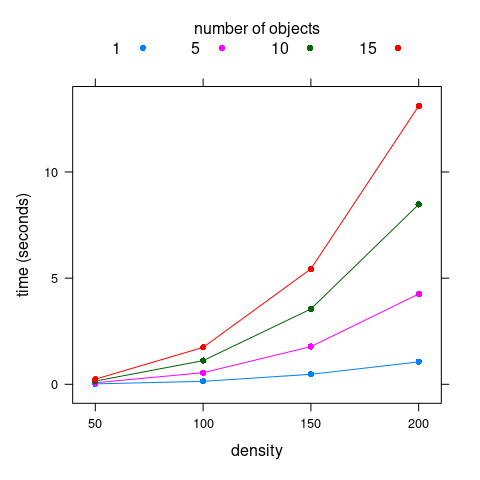### Related work

The HyperFun Project is not the only system that provides CSG and/or FRep. However, because the HyperFun source is distributed as part of the 'hyperfun' package, and because it is relatively easy to translate R expressions (like hfSphere()) into the HyperFun language and to integrate R expressions with the HyperFun language (via the hfR() function), the 'hyperfun' package provides a nice low-dependency, high-level interface for working with CSG and FRep in R.

Several R packages provide interfaces to much larger and more complex 3D graphics systems, though these tend to be lower level and even if they provide CSG they do not support FRep. For example, the 'rgl' package provides an interface to OpenGL and the 'Rvcg' package (Schlager, 2017) provides an interface to the VCG computer graphics library. There are also several packages that provide at least limited interfaces to the CGAL graphics library: 'cgalMesh' (Laurent, 2022a), 'MeshesTools' (Laurent, 2022b), and 'PolygonSoup' (Laurent, 2022c).

There are other computer graphics libraries that provide both CSG and FRep, for example, libfive and ImplicitCAD. These are both more modern than HyperFun and creating an R interface could be worth exploring, particularly for ImplicitCAD; an interface for libfive may require more effort due to the lower-level nature of the library.

Another interesting package is 'isocubes' (mikefc, 2022). This is limited to isometric renderings of 3D cubes, but it includes the concept of signed distance fields (SDF; or signed distance functions), which appear to be FRep by another name.

### Future work

There are several areas where the 'hyperfun' package could be worked on further. For a start, there are still FRep library functions in HyperFun that have no R function counterpart, e.g., hfMetaball for describing metaballs (Nishimura et al., 1985) and hfSoft for describing soft objects (Wyvill et al., 1986). These absences also include functions for transforming objects such as translation and rotation, plus also stretching and twisting.

The HyperFun language also allows for specifying attributes of 3D objects, such as colours and material properties, but there is currently no way to access this from 'hyperfun'.

If the parallels between FRep and SDF hold true then it would be worthwhile exploring further transformations beyond what already exists in the FRep library, such as champfers and border grooves.

Finally, an interface to a more modern system like ImplicitCAD could be explored.

## 6. Technical requirements

The examples and discussion in this report relate to version 0.1-0 of the 'hyperfun' package.

This report was generated within a Docker container (see Resources section below).

## 7. Resources

• The raw source file for this report, a valid XML transformation of the source file, a 'knitr' document generated from the XML file, two R files and the bibtex file that are used to generate the table of contents and reference sections, two XSL files and an R file that are used to transform the XML to the 'knitr' document, and a Makefile that contains code for the other transformations and coordinates everything. These materials are also available on github.
• This report was generated within a Docker container. The Docker command to build the report is included in the Makefile above. The Docker image for the container is available from Docker Hub; alternatively, the image can be rebuilt from its Dockerfile.
• The script for the 3D diagram in the Applications Section.

## How to cite this report

Murrell, P. and Wong, J. (2022). "Constructive Solid Geometry and Function Representation in R" Technical Report 2022-04, Department of Statistics, The University of Auckland. Version 1. [ bib | DOI | http ]

## 8. References

Adzhiev, V., Cartwright, R., Fausett, E., Ossipov, A., Pasko, A., and Savchenko, V. (2002). Hyperfun project: A framework for collaborative multidimensional F-rep modeling. Implicit Surfaces '99, Eurographics/ACM SIGGRAPH Workshop. [ bib ]
[Cleveland, 1985]
Cleveland, W. S. (1985). The Elements of Graphing Data. Wadsworth Publ. Co., USA. [ bib ]
[Cleveland, 1993]
Cleveland, W. S. (1993 - 1993). Visualizing data. AT&T Bell Laboratories, Murray Hill, N.J. [ bib ]
[Genz and Bretz, 2009]
Genz, A. and Bretz, F. (2009). Computation of Multivariate Normal and t Probabilities. Lecture Notes in Statistics. Springer-Verlag, Heidelberg. [ bib ]
[Laurent, 2022a]
Laurent, S. (2022a). cgalMeshes: R6 Based Utilities for 3D Meshes using CGAL. R package version 1.0.0. [ bib | http ]
[Laurent, 2022b]
Laurent, S. (2022b). MeshesTools: Some Tools for 3D Meshes. R package version 1.0.0. [ bib | http ]
[Laurent, 2022c]
Laurent, S. (2022c). PolygonSoup: Mesh from Polygon Soup. R package version 1.0.1. [ bib | http ]
[mikefc, 2022]
mikefc (2022). Isometric Cube 3d Rendering Canvas. R package version 0.1.5. [ bib | http ]
Murdoch, D. and Adler, D. (2022). rgl: 3D Visualization Using OpenGL. R package version 0.109.6. [ bib | http ]
[Nishimura et al., 1985]
Nishimura, H., Hirai, M., Kawai, T., Kawata, T., Shirakawa, I., and Omura, K. (1985). Object modeling by distributed function and a method of image generation. Transactions of IECE of Japan, J68-D(4):718--725. [ bib ]
[R Core Team, 2022]
R Core Team (2022). R: A Language and Environment for Statistical Computing. R Foundation for Statistical Computing, Vienna, Austria. [ bib | http ]
[Schlager, 2017]
Schlager, S. (2017). Morpho and rvcg -- shape analysis in R. In Zheng, G., Li, S., and Szekely, G., editors, Statistical Shape and Deformation Analysis, pages 217--256. Academic Press. [ bib ]
[Ware, 2012]
Ware, C. (2012). Information Visualization: Perception for Design. Morgan Kaufmann, 3 edition. [ bib ]
[Wyvill et al., 1986]
Wyvill, G., McPheeters, C., and Wyvill, B. (1986). Data structure for soft objects. The Visual Computer, 2(4):227--234. [ bib ]